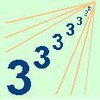# Indices and Surds### Climbing Powers

##### Age 16 to 18Challenge Level

$2\wedge 3\wedge 4$ could be $(2^3)^4$ or $2^{(3^4)}$. Does it make any difference? For both definitions, which is bigger: $r\wedge r\wedge r\wedge r\dots$ where the powers of $r$ go on for ever, or $(r^r)^r$, where $r$ is $\sqrt{2}$?### The Root of the Problem

##### Age 14 to 18Challenge Level

Find the sum of this series of surds.### Power Stack

##### Age 16 to 18 ShortChallenge Level

When you stack powers, how do you evaluate them?### Quick Sum

##### Age 16 to 18 ShortChallenge Level

Is this surd sum exactly 3?### Irrational Arithmagons

##### Age 16 to 18Challenge Level

Can you work out the irrational numbers that belong in the circles to make the multiplication arithmagon correct?